AP Calculus BC : Ratio Test and Comparing Series

Example Questions

1 3 Next →

Example Question #2 : Ratio Test

Assuming that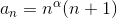,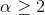. Using the ratio test, what can we say about the series: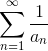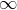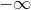We cannot conclude when we use the ratio test.

It is convergent.We cannot conclude when we use the ratio test.

Explanation:

As required by this question we will have to use the ratio test.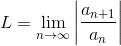if L<1 the series converges absolutely, L>1 the series diverges, and if L=1 the series could either converge or diverge.

To do so, we will need to compute :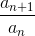. In our case: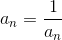Therefore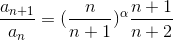.

We know that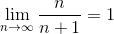This means that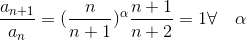Since L=1 by the ratio test, we can't conclude about the convergence of the series.

Example Question #4 : Ratio Test

Using the ratio test,

what can we say about the series.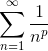whereis an integer that satisfies: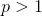We can't use the ratio test to study this series.

We can't conclude when we use the ratio test.We can't conclude when we use the ratio test.

Explanation:

Let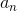be the general term of the series. We will use the ratio test to check the convergence of the series.

The Ratio Test states: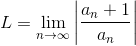then if,

1) L<1 the series converges absolutely.

2) L>1 the series diverges.

3) L=1 the series either converges or diverges.

Therefore we need to evaluate,we have,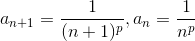therefore: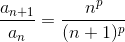.

We know thatand therefore,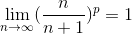This means that :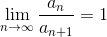By the ratio test we can't conclude about the nature of the series. We will have to use another test.

Example Question #5 : Ratio Test

Consider the following series :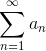whereis given by: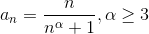. Using the ratio test, find the nature of the series.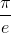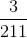We can't conclude when using the ratio test.

The series is convergent.

We can't conclude when using the ratio test.

Explanation:

Letbe the general term of the series. We will use the ratio test to check the convergence of the series.if L<1 the series converges absolutely, L>1 the series diverges, and if L=1 the series could either converge or diverge.

We need to evaluate,we have: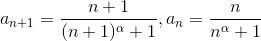.

Therefore: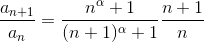. We know that,and therefore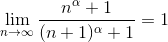This means that :.

By the ratio test we can't conclude about the nature of the series. We will have to use another test.

Example Question #13 : Convergence And Divergence

We consider the series,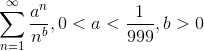.

Using the ratio test, what can we conclude about the nature of convergence of this series?

We can't use the ratio test here.

We will need to know the values ofto decide.

The series converges to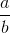.

The series is convergent.

The series is divergent.

The series is convergent.

Explanation:

Note that the series is positive.

As it is required we will use the ratio test to check for the nature of the series.

We have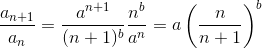.

Therefore,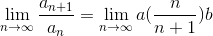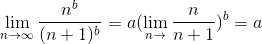if L>1 the series diverges, if L<1 the series converges absolutely, and if L=1 the series may either converge or diverge.

Since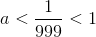the ratio test concludes that the series converges absolutely.

Example Question #21 : Ratio Test And Comparing Series

Use the ratio test to determine if the series diverges or converges: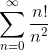The series diverges.

Unable to determine.

The series converges.

The series diverges.

Explanation: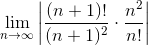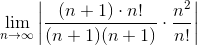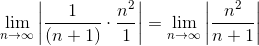This limit is infinite, so the series diverges.

Example Question #22 : Ratio Test And Comparing Series

Use the ratio test to determine if this series diverges or converges: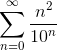The series diverges

The series converges

Unable to determine

The series converges

Explanation: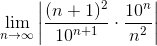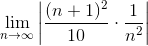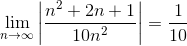Since the limit is less than 1, the series converges.

Example Question #23 : Ratio Test And Comparing Series

Use the ratio test to determine if the series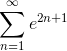converges or diverges.

Unable to determine

The series diverges.

The series converges.

The series diverges.

Explanation: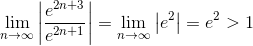The series diverges.

Example Question #24 : Ratio Test And Comparing Series

Use the ratio test to determine if the series diverges or converges: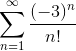The series diverges.

The series converges.

Unable to determine

The series converges.

Explanation: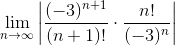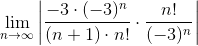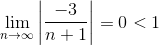The series converges.

1 3 Next →